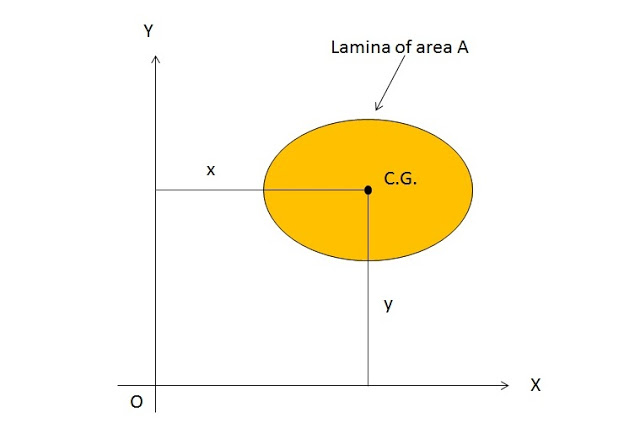# What is First Moment of Area?

In this article, we will discuss about the definition of the first moment of area or moment of area, application, and example. We will also derive the formula for it.

## First Moment of Area

Considered a thick lamina or a body having area A. let x is the distance of C.G. of the area from the axis OY and y is the distance of C.G. of the area from the axis OX.Here

x = Distance of C.G. of area A from axis OY

y = Distance of C.G. of area A from axis OX

Then,This equation (1) is known as the first moment of area about the axis OY

SimilarlyThis Equation (2) is called the first moment of area about the axis OX.

### Conclusion:

#### First moment of area/moment of area:

The first moment of area of a lamina about an axis is defined as the product of area of the lamina and the perpendicular distance of the C.G. of the area form the axis.

### Application

It is used to determine the center of gravity of area.

### Numerical Example

If we have a lamina having area 10 cm2 and the distance of its C.G from the axis are shown in
the figure given below.

Then

• The first moment of area about axis OY = 10 x 5 = 50
• The first moment of area about axis OX = 10 x 7 = 70

Here we have discussed the what is the first moment of area, its formula, and application with numerical problems. If you found this piece of information useful then don’t forget to like, share, and subscribe to us.